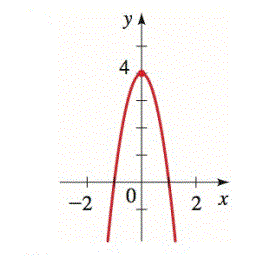Chapter 12.4, Problem 29E### Algebra and Trigonometry (MindTap ...

4th Edition
James Stewart + 2 others
ISBN: 9781305071742

#### Solutions

Chapter
Section### Algebra and Trigonometry (MindTap ...

4th Edition
James Stewart + 2 others
ISBN: 9781305071742
Textbook Problem

# 29-34 ■Finding the Equation of Shifted Conic Find an equation for the conic whose graph is shown.To determine

To find:

An equation for the conic whose graph is shown.

Explanation

Given:

The graph

Approach:

From given graph one can find out vertex and the point of intersection with x-axis. Then the standard equation of shifted parabola facing up down can be used

(xh)2=4p(yk)

Calculation:

We can find the vertex (h,k) from the graph and its value is (0,4), so h=0 and k=4.

Now by putting the value of (h,k) in the standard equation of shifted parabola facing downward

(x0)2=4p(y4)

x2=4p(y4)

Above equation must satisfy the point of intersection of graph with x-axis

### Still sussing out bartleby?

Check out a sample textbook solution.

See a sample solution

#### The Solution to Your Study Problems

Bartleby provides explanations to thousands of textbook problems written by our experts, many with advanced degrees!

Get Started